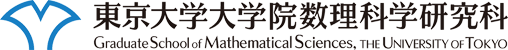このページに含まれる映像は自由に視聴することができますが、許可無くコピー・配布などの行為を禁止します。

# 数理談話会

### 講演者

Yves Benoist 氏 ( CNRS, Paris-Sud)

### 講演題目

Arithmeticity of discrete subgroups (英語)

### 講演概要

By a theorem of Borel and Harish-Chandra,
an arithmetic group in a semisimple Lie group is a lattice.
Conversely, by a celebrated theorem of Margulis,
in a higher rank semisimple Lie group G
any irreducible lattice is an arithmetic group.

The aim of this lecture is to survey an
arithmeticity criterium for discrete subgroups
which are not assumed to be lattices.
This criterium, obtained with Miquel,
generalizes works of Selberg and Hee Oh
and solves a conjecture of Margulis. It says:
a discrete irreducible Zariski-dense subgroup
of G that intersects cocompactly at least one
horospherical subgroup of G is an arithmetic group.